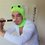# Tough problems on Calculus.

Here are some problems in calculus which i am not being able to solve:

$1.$ Evaluate: $\large{\lim\limits_{n \to \infty}\frac{1}{2n}log\binom{2n}{n}}$

$2.$ Consider the following sequence of positive integers regarding ${a_i}$

$a_1=1$ and $a_n=n(a_{n-1}+1)$ for all $n≥2$.

Calculate: $\large{\lim\limits_{n \to \infty}(1+\frac{1}{a_1})(1+\frac{1}{a_2})\dots(1+\frac{1}{a_n})}$

$3.$Let $f:\mathbb{R}\to \mathbb{R}$ be function that is differentiable $n+1$ times for some positive integer $n$. The $i^{th}$ derivative is denoted by $f^{i}$. Suppose:

$f(1)=f(0)=f^{1}(0)=f^{2}(0)=\dots=f^{n}(0)=0$

Prove that: $\large{f^{n+1}(x)=0}$

$4.$Let $f$ be a real valued differentiable function on the real line $\mathbb{R}$ such that $\large{\lim\limits_{x\to 0}\frac{f(x)}{x^{2}}}$ exists, and is finite. prove that: $\large{f'(0)=0}$Note by Aritra Jana
5 years, 7 months ago

This discussion board is a place to discuss our Daily Challenges and the math and science related to those challenges. Explanations are more than just a solution — they should explain the steps and thinking strategies that you used to obtain the solution. Comments should further the discussion of math and science.

When posting on Brilliant:

• Use the emojis to react to an explanation, whether you're congratulating a job well done , or just really confused .
• Ask specific questions about the challenge or the steps in somebody's explanation. Well-posed questions can add a lot to the discussion, but posting "I don't understand!" doesn't help anyone.
• Try to contribute something new to the discussion, whether it is an extension, generalization or other idea related to the challenge.

MarkdownAppears as
*italics* or _italics_ italics
**bold** or __bold__ bold
- bulleted- list
• bulleted
• list
1. numbered2. list
1. numbered
2. list
Note: you must add a full line of space before and after lists for them to show up correctly
paragraph 1paragraph 2

paragraph 1

paragraph 2

[example link](https://brilliant.org)example link
> This is a quote
This is a quote
    # I indented these lines
# 4 spaces, and now they show
# up as a code block.

print "hello world"
# I indented these lines
# 4 spaces, and now they show
# up as a code block.

print "hello world"
MathAppears as
Remember to wrap math in $$ ... $$ or $ ... $ to ensure proper formatting.
2 \times 3 $2 \times 3$
2^{34} $2^{34}$
a_{i-1} $a_{i-1}$
\frac{2}{3} $\frac{2}{3}$
\sqrt{2} $\sqrt{2}$
\sum_{i=1}^3 $\sum_{i=1}^3$
\sin \theta $\sin \theta$
\boxed{123} $\boxed{123}$

Sort by:

1. Use the Stirling's approximation $n!\sim \sqrt{2\pi n}\bigg(\frac{n}{e}\bigg)^n$

- 5 years, 7 months ago

3: I don't know if this is a valid proof:

$f^{(n+1)}(x) = 0$ iff $f^{(n)}(x) = C_{n}$ where $C_{i}$ are some arbitrary constants. However, $f^{(n)}(0) = 0$; because $f^{(n)}(x) = f^{(n)}(0) = 0$ for all $x \in \mathbb{R}$, therefore, $f^{(n)}(x) = C_{n} = 0$.

Repeating the same argument for $n-1$, $n-2$, and so on, we see that it must be true that $f(x) = 0$ and hence $f^{(n+1)}(x) = 0$.

Logically it seems sound:

$\forall n \in \mathbb{Z}, x \in \mathbb{R} \Bigg(\boxed{f^{(n+1)}(x) = 0 \wedge f^{(n)}(0) = 0} \longleftrightarrow \boxed{f^{(n)}(x) = C_{n} \wedge f^{(n)}(0) = 0} \longleftrightarrow \boxed{f^{(n)}(x) = 0} \Bigg)$

- 5 years, 7 months ago

Using PMI

- 5 years, 7 months ago

What's PMI?

- 5 years, 7 months ago

Principle of mathematical induction

- 5 years, 7 months ago

@U Z Yeah, it's pretty much induction but in reverse with base case $n+1$.

- 5 years, 7 months ago

@megh choksi @Krishna Sharma @Sandeep Bhardwaj ..any idea as to how to approach the 3rd one?

- 5 years, 7 months ago

I posted a solution just now, and I think it works. It seems too easy though, which is troubling :<

- 5 years, 7 months ago

2) $lim_{n \to \infty} ( \dfrac{a_{1} + 1}{a_{1}}\dfrac{a_{2} + 1}{a_{2}}...\dfrac{a_{n} + 1}{a_{n}}$

$a_{n-1} - 1 = \dfrac{a_{n}}{n}$

Thus,

$lim_{n \to \infty} (\dfrac{a_{2}}{2}\dfrac{a_{3}}{3}....\dfrac{a_{n+1}}{n+1}.\dfrac{1}{a_{1}a_{2}...a_{n}}$

$= lim_{n \to \infty} \dfrac{a_{n+1}}{(n+1)!} = lim_{n \to \infty}\dfrac{1+a_{n}}{n!}$

$= lim_{n \to \infty} \dfrac{1}{n!} + \dfrac{a_{n}}{n!} = lim_{n \to \infty} \dfrac{1}{n!} + \dfrac{1}{(n-1)!} \dfrac{a_{n -1}}{(n-1)!}$

$lim_{n \to \infty} \dfrac{1}{n!} + \dfrac{1}{(n-1)!} + \dfrac{1}{(n-2)!} + .... \dfrac{1}{(1)!} + \dfrac{a_{1}}{(1)!}$

$= e$ as $a_{1} = 1$

- 5 years, 7 months ago

Great :D

- 5 years, 7 months ago

SUPERB! :D

- 5 years, 7 months ago

In 1st use limit as a sum

We will first cancel one $n!$

Now it becomes

$\displaystyle lim_{n \to \infty } \frac{1}{n} log\left( \dfrac{(n+1)(n+2)....(n+n)}{1.2.3...n} \right)$

$\displaystyle lim_{n \to \infty } \frac{1}{2n} \sum_{r = 1}^{n} log \left( \dfrac{n + r}{r} \right)$

Changing summation into integration

$\displaystyle \frac{1}{2} \int_0^{1} log\left( \dfrac{1+x}{x} \right) dx$

Now this can be solved easily

4th is easy if limit exist then it is $\dfrac{0}{0}$ form Applying L-hospital $\dfrac{f'(x)}{2x}$ so this should also be $\dfrac{0}{0}$ because limit exist hence

$f'(0) = 0$

2nd looks interesting I'll try it

- 5 years, 7 months ago

for the 4th one.. that is exactly what i thought.. but i was confused that it might be incorrect.. are you sure that method is right?

- 5 years, 7 months ago

Yes :), what's the answer to 2nd?

- 5 years, 7 months ago

the answer given is $\LARGE{\boxed{e}}$

- 5 years, 7 months ago